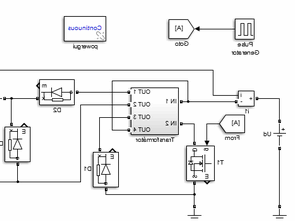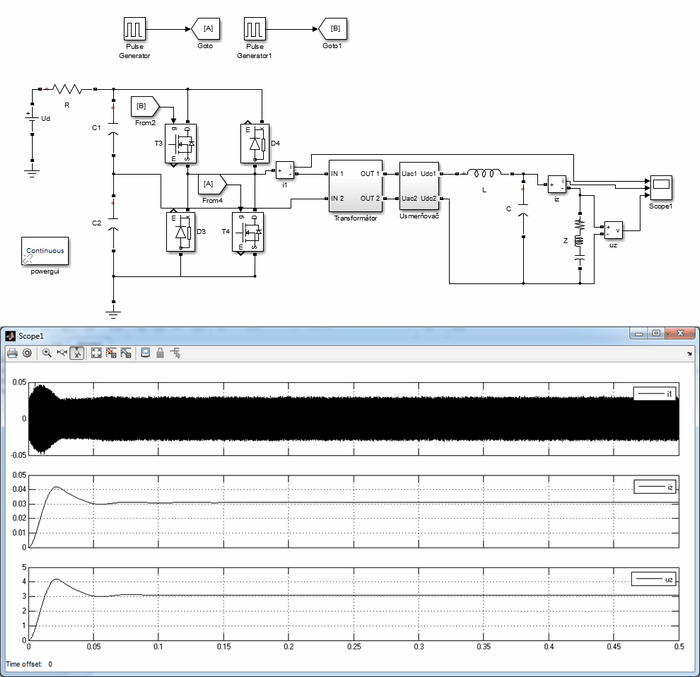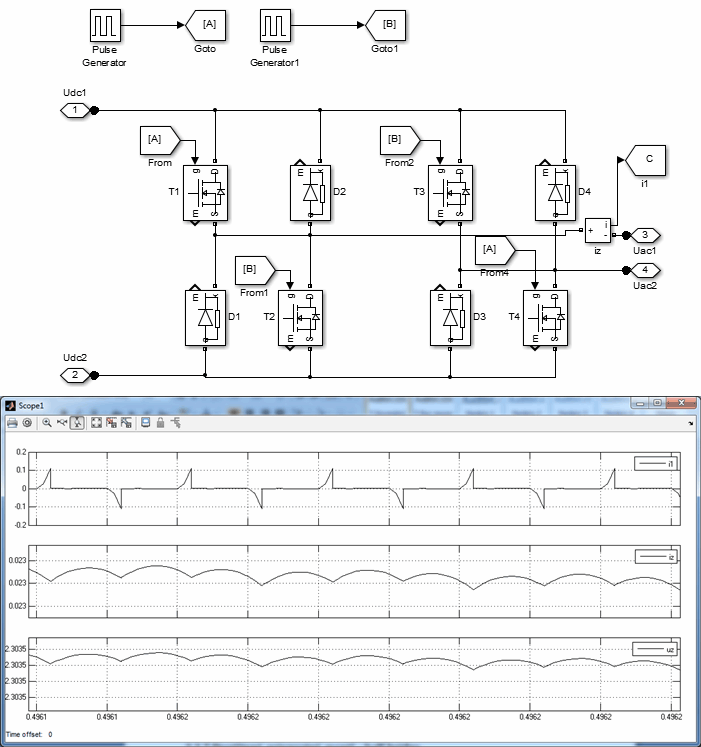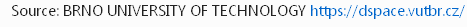# DC DC Converter Models Matlab

| September 19, 2021 UpdatedMatlab models of DC DC converters A study on standard DC DC converters with transformers, their applications and simulation results in Matlab Simulink software environment using Simscape library. A large part of the work is devoted to the theory and design of the transformer model, which is an important part of the transformer DC DC converter model.

The transformer model is based on theoretical data and its functionality has been validated on the basis of a reference model. The main part of the work focused on clarifying the principle of operation of various DC DC converters with a transformer, then their modeling in the Matlab Simulink program, simulation and processing of simulation results.Validation of functionality of Matlab Transformer model
Matlab model of a three-winding transformer
Current and voltage stabilization operation for the load of a single-acting open-loop converter
Parameters for Matlab model of single-effect transducer with demagnetizing winding
Current and voltage stabilization process at the load of a single-acting open-loop converter
Single-acting direct-pass converter model with demagnetization to a zener diode
Model parameters of the single-effect converter with demagnetization to the zener diode
Current and voltage stabilization operation for the load of a single-acting open-loop converter
Model parameters of the feedback pass transducer
Matlab model of two single-acting permeable backpressure transducers working until the last moment
Current and voltage stabilization process at load of two single-acting transmissions
Current and voltage stabilization process at load of two single-acting transmissions
Model parameters of the feedback pass transducer
Model parameters of double-acting open-loop converter
Model parameters of double-acting open-loop converter
Matlab model of double-acting pass-through converter with two-way node rectifier
double-acting open-loop converter
Double-acting open-loop converter model with current doubler
Current and voltage profile for load in a double-acting open-circuit converter with current
Parameters of the half-bridge transducer model
Double-acting pass-through converter Matlab model – half bridge
Current and voltage profile at load with double-acting open-loop converter – half bridge
Parameters of the double-acting pass-through converter model – push-pull
Matlab model parameters of single-acting blocking transducer
Current and voltage profile of a single-acting blocking converter
Detail of the course of current and voltage in the load of a single-acting blocking converter